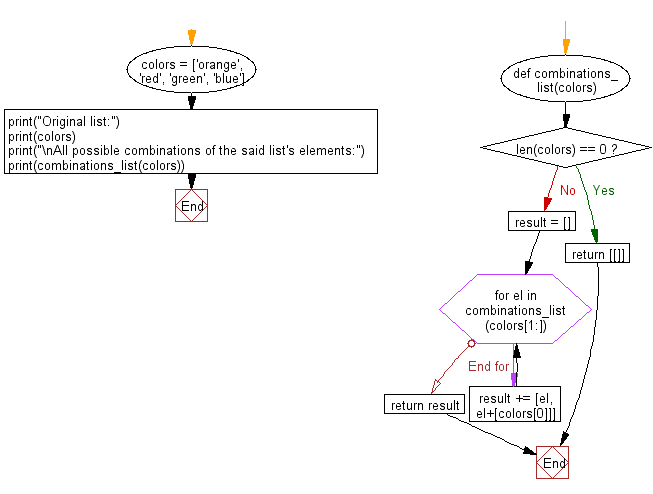﻿ Python: All possible combinations of the elements of a given list - w3resource# Python: All possible combinations of the elements of a given list

## Python List: Exercise - 149 with Solution

Write a Python program to get all possible combinations of the elements of a given list.

Sample Solution:

Python Code:

``````def combinations_list(colors):
if len(colors) == 0:
return [[]]
result = []
for el in combinations_list(colors[1:]):
result += [el, el+[colors]]
return result
colors = ['orange', 'red', 'green', 'blue']
print("Original list:")
print(colors)
print("\nAll possible combinations of the said list’s elements:")
print(combinations_list(colors))
```
```

Sample Output:

```Original list:
['orange', 'red', 'green', 'blue']

All possible combinations of the said list's elements:
[[], ['orange'], ['red'], ['red', 'orange'], ['green'], ['green', 'orange'], ['green', 'red'], ['green', 'red', 'orange'], ['blue'], ['blue', 'orange'], ['blue', 'red'], ['blue', 'red', 'orange'], ['blue', 'green'], ['blue', 'green', 'orange'], ['blue', 'green', 'red'], ['blue', 'green', 'red', 'orange']]
```

Flowchart:## Visualize Python code execution:

The following tool visualize what the computer is doing step-by-step as it executes the said program:

Python Code Editor:

Have another way to solve this solution? Contribute your code (and comments) through Disqus.

What is the difficulty level of this exercise?

Test your Python skills with w3resource's quiz

﻿

## Python: Tips of the Day

Floor Division:

When we speak of division we normally mean (/) float division operator, this will give a precise result in float format with decimals.

For a rounded integer result there is (//) floor division operator in Python. Floor division will only give integer results that are round numbers.

```print(1000 // 300)
print(1000 / 300)```

Output:

```3
3.3333333333333335```